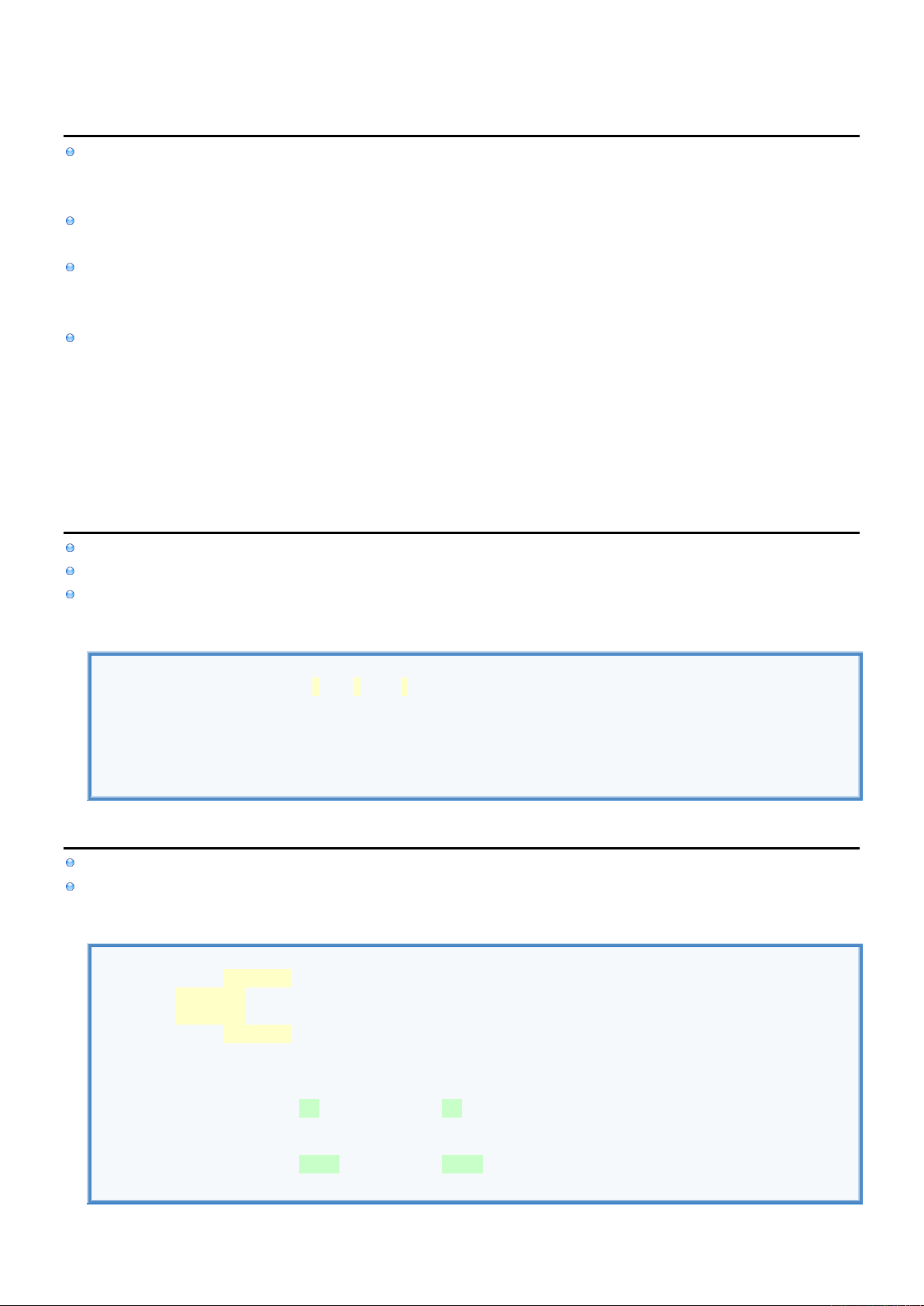2
2
.
.
2
2
.
.
5
5
D
D
a
a
t
t
a
a
T
T
y
y
p
p
e
e
-
-
G
G
e
e
n
n
e
e
r
r
i
i
c
c
I
I
n
n
f
f
o
o
Generic Data Types allow you to write Functions, Classes, Structures & Enumerators that work with any Data Type.
Optionally you can use Constraints to specify subset of applicable Data Types.
This allows you to write single Method/Class/Struct that accepts different Data Types for their Properties and Parameters.
With specific Data Types you say which Data Type goes into which Variables all the time.
With Generic Data Types you say which Data Type goes into which Variable for each Method Call or Class/Struct Instance
This allows you to create a single Method/Class/Struct that can perform addition on two Parameters where these two
Parameters can be of any common Data Type specified when Method is called or Instance is created. Instead of create a
separate Method/Class/Struct for adding: Integers, Floats, Doubles.
This avoids code duplication by avoiding to write
Multiple Functions that have the same body but different Data Types of Input Parameters.
Instead you write single Function that uses Generic Data Types instead of specific Data Types for Input Parameters.
These Generic Data Types are then converted into specific Data Types during function call.
Multiple Classes that have the same body but different Data Types for Properties or Method's Input Parameters.
Instead you write single Class that uses Generic Data Types instead of specific Data Types
These Generic Data Types are then converted into specific Data Types when instance is created.
G
G
e
e
n
n
e
e
r
r
i
i
c
c
F
F
u
u
n
n
c
c
t
t
i
i
o
o
n
n
w
w
i
i
t
t
h
h
C
C
o
o
n
n
s
s
t
t
r
r
a
a
i
i
n
n
t
t
[
[
R
R
]
]
In this example we use Numeric Constraint to say that Generic Data Type can only be Numeric Data Type.
When calling Generic Method you don't explicitly specify which Data Types to use for Generic Data Types.
Instead Swift will figure that out on its own based on the Input Parameters Data Types.
Generic Function with Constraint
//DECLARE GENERIC FUNCTION.
func addNumbers<T: Numeric>(x: T, y: T) -> (T) { return x + y }
//USE FUNCTION
var result1 = addNumbers(x: 10, y:20) //Use Integeres
var result2 = addNumbers(x: 10.5, y:20.5) //Use Doubles
print(result1)
G
G
e
e
n
n
e
e
r
r
i
i
c
c
S
S
t
t
r
r
u
u
c
c
t
t
w
w
i
i
t
t
h
h
C
C
o
o
n
n
s
s
t
t
r
r
a
a
i
i
n
n
t
t
[
[
R
R
]
]
In this example we use Numeric Constraint to say that Generic Data Type can only be Numeric Data Type.
This allows us to use "+" Operator which otherwise wouldn't work without overloading it.
Generic Struct with Constraint
//DECLARE GENERIC STRUCT.